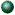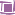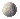## An Elementary Introduction to Elliptic functions

This course will focus on a topic typically covered in a second course in complex analysis: Elliptic function theory. Elliptic functions  as developed by Jacobi, Weierstrass, Eisenstein, Dedekind, and others  are one of the crowning achievements of 19th century mathematics and are widely applied in physics and engineering. Their study is the natural continuation of the analysis of polynomial, exponential, and trigonometric functions of a complex variable. In the 20th century, the analysis of the beautiful transformation properties of elliptic functions developed into the theory of elliptic curves and modular forms, a central topic of algebraic geometry and number theory. Recently, elliptic functions have played an important role in the 21st century mathematics inspired by theoretical physics.

Here are some topics from the course:Arithmetic-Geometric Mean (AGM) and elliptic integrals;Doubly periodic q-functions  Jacobi;The Weierstrass P-function;Applications to PDEs (maximal surfaces equation) and number theory (representing integers as sums of squares and quadratic forms).

### LiteratureAkheizer, N.I.Providence, RI: Amer. Math. Soc., 1990.Chandrasekharan, K.Berlin: Springer-Verlag, 1985.Hille, E. Analytic Function Theory, Vol. II. Ginn & Co., 1962.

here

TBA

### Other InformationHere you can find the list of basic and advanced books in Elliptic Functions and the related topicsHere is the Wolfram Research  documentation concerning Elliptic functions and Elliptic Integrals for the Mathematica usersThe list of the Jacobi Elliptic Functions formulae is hereApplications: Audio Synthesis by Means of Elliptic Functions due to Vittorio Cafagna and Filippo D'ElisoThis may also be useful JBack to Homepage